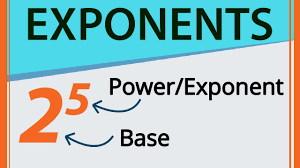# Exponents and Powers: Repeated Multiplication of the Same Number

In this quiz, you will find questions related to the exponents and powers, their properties, etc.Start Quiz

If 2^3 × 2^4 = 2^?, then ? =

3 4 1 7

(-1)^even number =

-1 1 0 none of these

What is the base in 8^2?

8 2 6 10

The exponential form of 625 is

5^2 5^3 5^4 5^5

The exponential form of 81 is

3^4 3^3 3^2 none of these

Express 729 as a power of 3.

9^3 3^4 3^6 3^2

The short notation 10^4 stands for the product.

10 × 10 × 10 × 10 4 × 10 10 + 10 + 10 + 10 None of these

Find the number from the following expanded forms 3 × 10^4 + 7 × 10^2 + 5 × 10^0.

375 30705 3705 None of these

The value of (−1)^47 is

-1 1 0 None of these

Find 7^3 ÷ 7^3.

7^6 7^9 7^0 None of these

(-6)^3 =

-216 216 18 -18

10^12 ÷ 10^9 is equal to

10^3

10^-3

10^21

None of all

The value of (5^30×5^20) ÷ (5^4)^5 is

5^30

5^20

5^50

None of all

3^5 =

243

234

15

35

(-5)^2 × 3 =

-75

75

-30

30

Quiz/Test Summary
Title: Exponents and Powers: Repeated Multiplication of the Same Number
Questions: 15
Contributed by: